Global
• Global
• Türkiye
• Việt Nam
• México
• Perú
• Colombia
• Argentina
• Brasil
• India
• ประเทศไทย
• Indonesia
• Malaysia
• Philippines
• Deutschland
• 中國香港
• 中國台灣
• السعودية
• مصر
• پاکستان
• Россия
• 日本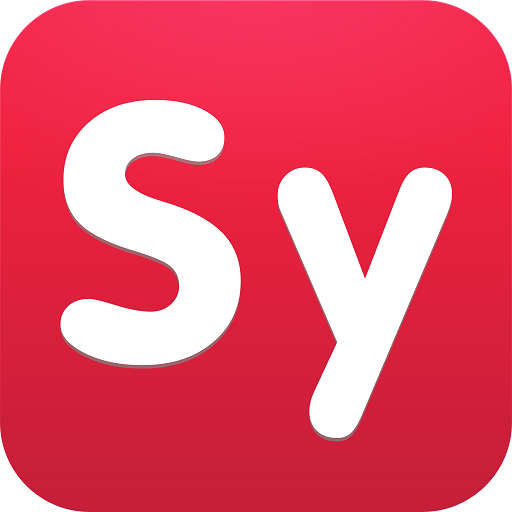## Symbolab: Math Solver & Helper PC

4.5Version: 9.6.15

Symbolab

# Download Symbolab: Math Solver & Helper on PC With GameLoop Emulator

Symbolab: Math Solver & Helper, coming from the developer Symbolab, is running on Android systerm in the past.

Now, You can play Symbolab: Math Solver & Helper on PC with GameLoop smoothly.

Download it in the GameLoop library or search results. No more eyeing the battery or frustrating calls at the wrong time any more.

Just enjoy Symbolab: Math Solver & Helper PC on the large screen for free！

### Symbolab: Math Solver & Helper Introduction

Symbolab Math Solver & Helper is a new, professional math calculator and math problem solver. Use Symbolab’s photo math scanner to answer questions, get some help with your math homework, or solve math problems with step-by-step solutions. Have a private math tutor in your pocket!

Symbolab is used by over 200 million students to work through and understand more than one billion math problems and explanations. Take a photo with our math answer scanner to learn and solve equations, integrals, derivatives, trigonometry, geometry, calculus problems, limits, and much more.

Symbolab Math Solver & Helper is composed of over five hundred of Symbolab's most powerful math calculators:

Calculus calculator

Graphing Calculator

Fraction Calculator

Equation Calculator, including differential equation

Integral Calculator

Derivative Calculator

Limit calculator

Inequality Calculator

Trigonometry Calculator

Matrix Calculator

Functions Calculator

Series Calculator

ODE Calculator

Laplace Transform Calculator

Download Symbolab Math solver & Helper to experience the full set of Symbolab calculators and get some help with our unique math helper. Symbolab Math Solver & Homework Helper can guide you step by step on how to solve a diverse range of math problems, including Pre Algebra, Algebra, Pre Calculus, Calculus, Trigonometry, Geometry, Functions, Matrix, Vectors, and Statistics. Simply scan a math problem with a math answer scanner to get started!

You can also use Symbolab’s photo math scanner as a math word problem solver: whether its a Calculus word problem, an Algebra word problem, Geometry or Trigonometry word problems, and so on - Symbolab is the math homework helper that can take your homework answers to the next level and guide all the way through the solution. Learn and understand mathematics in a new, efficient way to expand your horizons and deepen your knowledge.

Symbolab is a global leader in education technology, with over 200 million users worldwide. Symbolab is committed to helping students learn mathematics, providing step-by-step math answers to any math problem, including AI-driven personalized learning, assessments, insights, and more.

Symbolab solves any problem for free. In order to view step-by-step solutions, you can subscribe weekly, monthly, or yearly.

Payment will be charged to iTunes Account at confirmation of purchase.

Subscription automatically renews unless auto-renew is turned off at least 24-hours before the end of the current period.

Account will be charged for renewal within 24 hours prior to the end of the current period, and identify the cost of the renewal.

Subscriptions may be managed by the user, and auto-renewal may be turned off by going to the user's Account Settings after purchase.

### Preview

•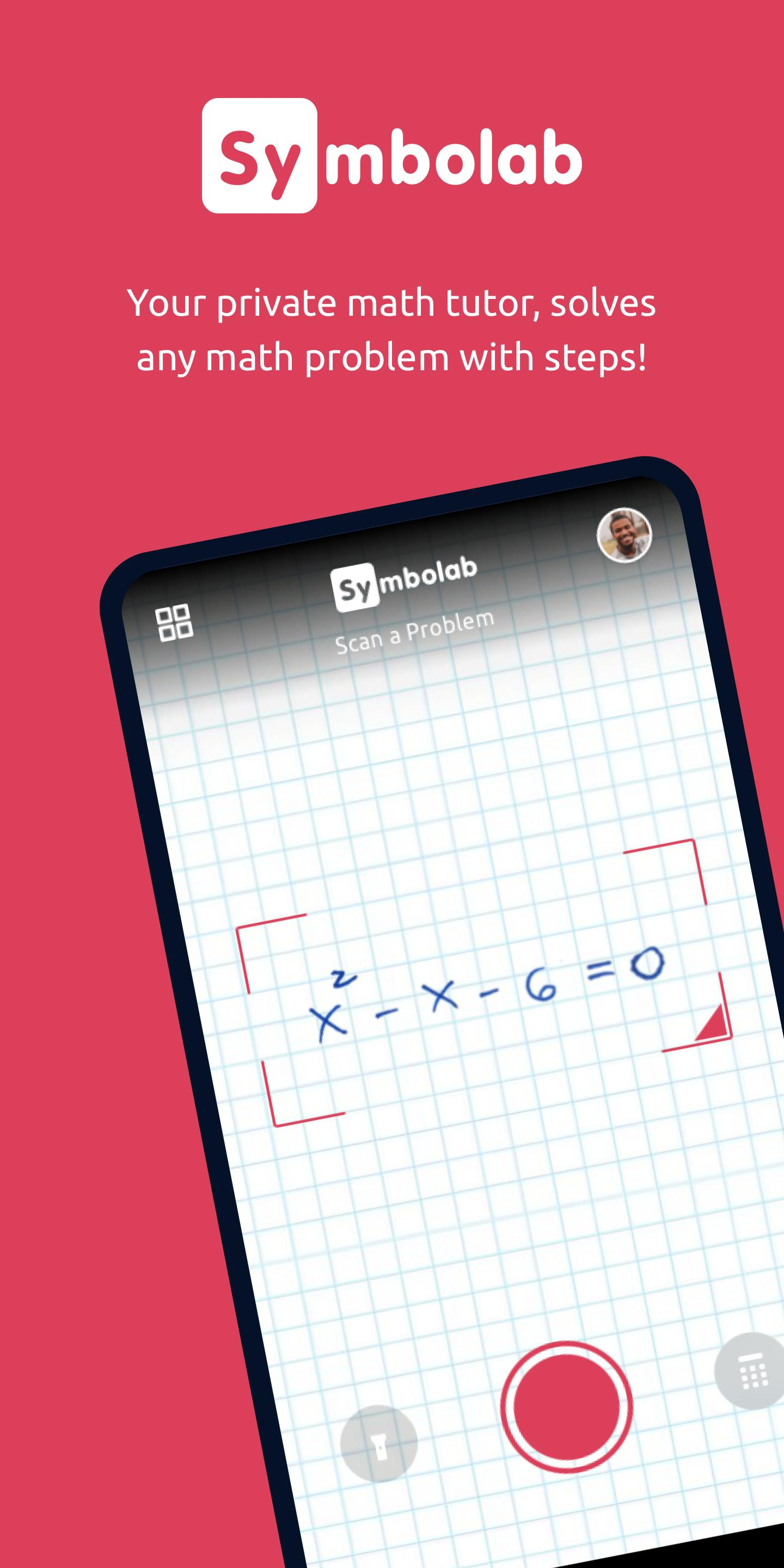•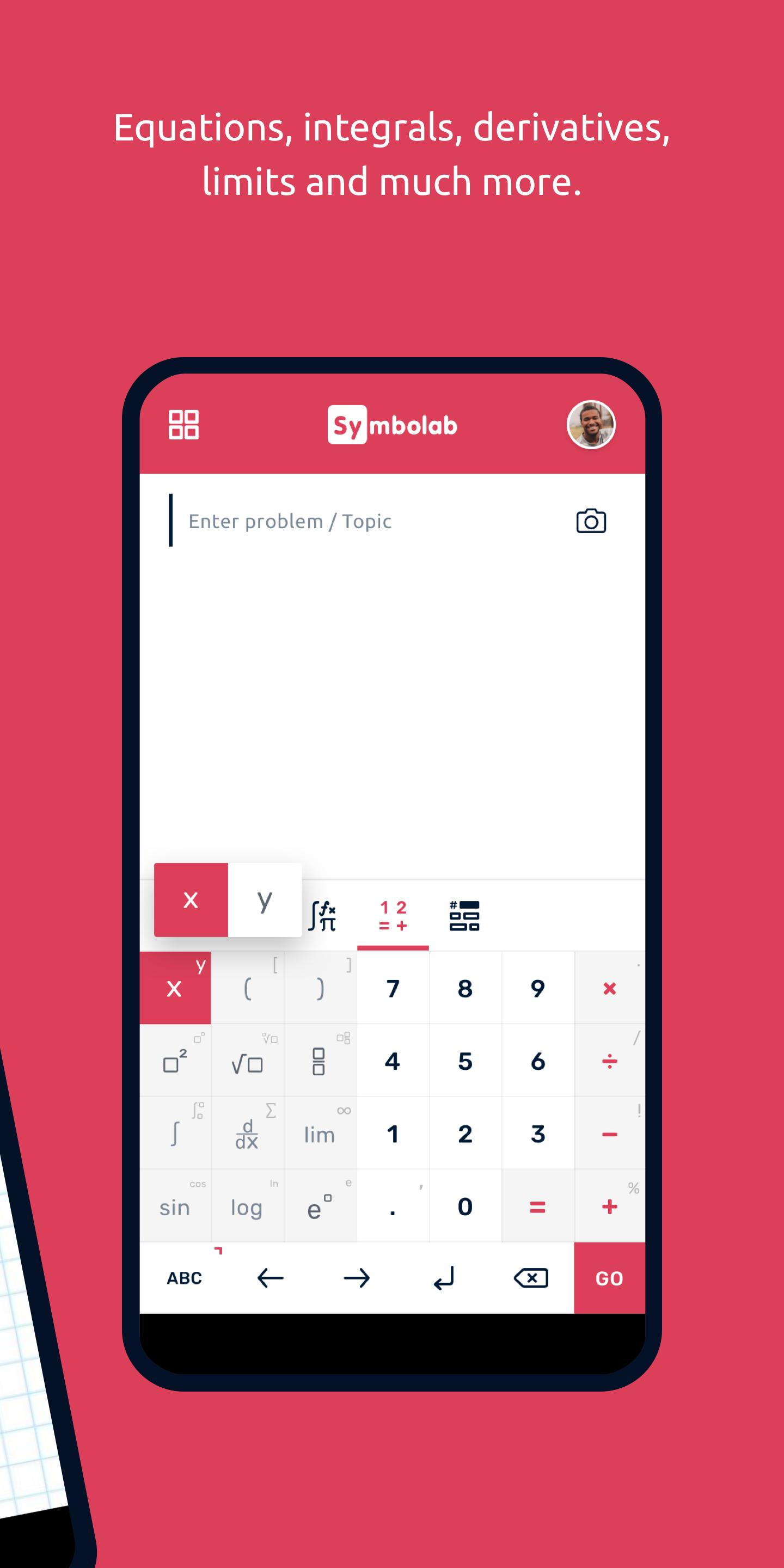•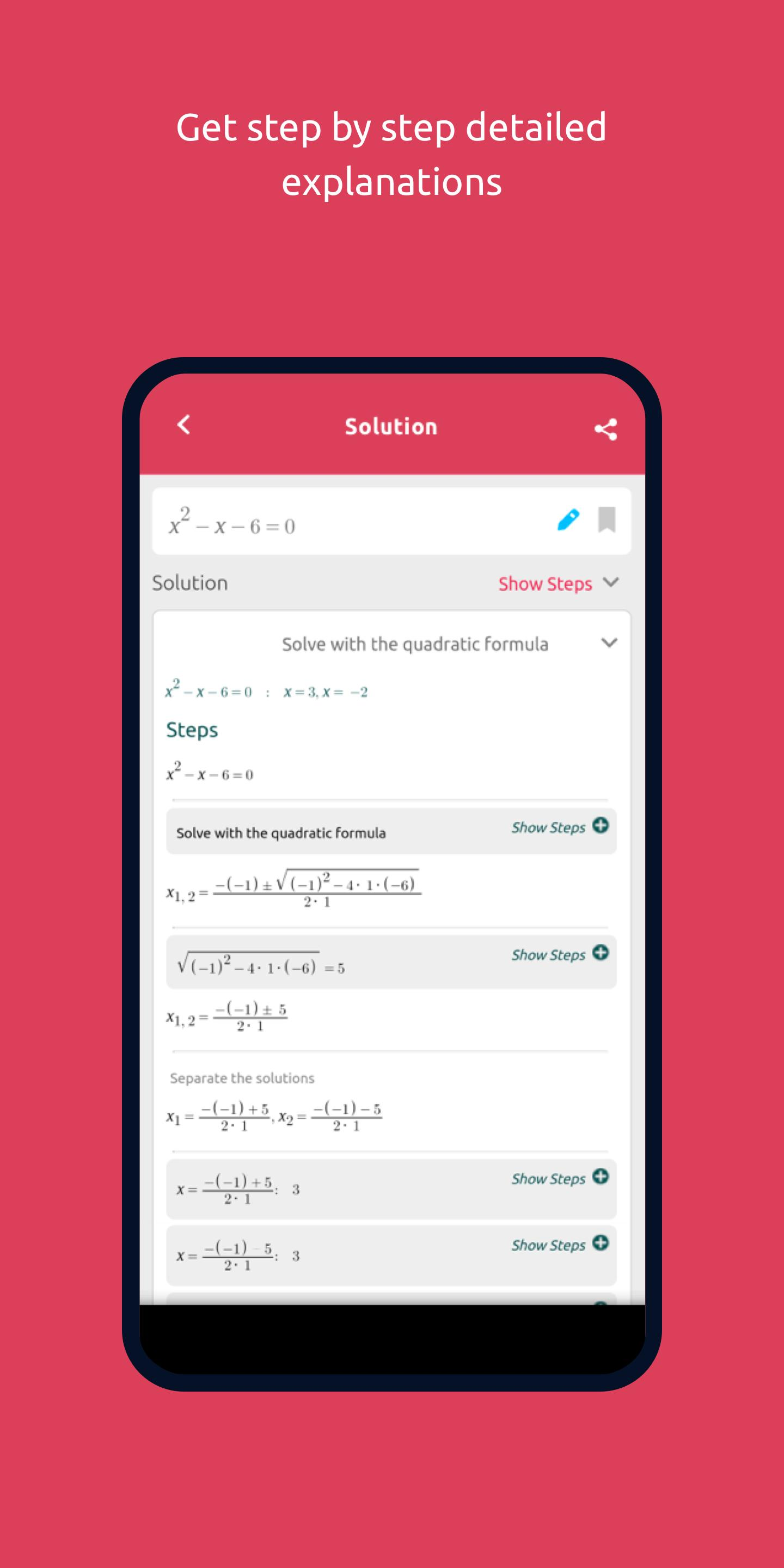•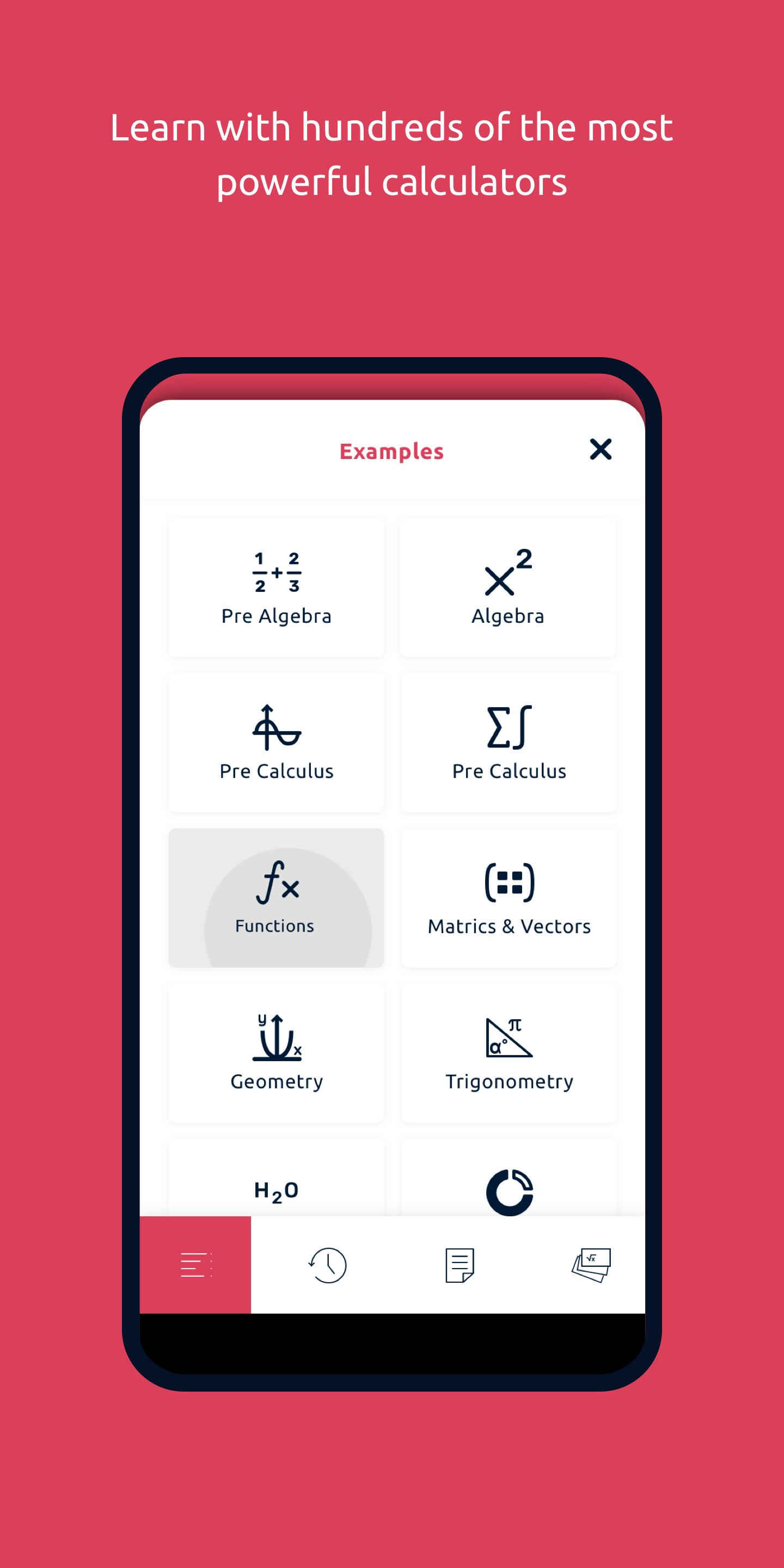• Developer

Symbolab

9.6.15

• Last Updated

2022-09-12

• Category

education

• Available on

## How to play Symbolab: Math Solver & Helper with GameLoop on PC

1. Download GameLoop from the official website, then run the exe file to install GameLoop

2. Open GameLoop and search for “Symbolab: Math Solver & Helper” , find Symbolab: Math Solver & Helper in the search results and click “Install”

3. Enjoy playing Symbolab: Math Solver & Helper on GameLoop

## Minimum requirements

OS

Windows 8.1 64-bit or Windows 10 64-bit

GPU

GTX 1050

CPU

i3-8300

Memory

8GB RAM

Storage

1GB available space

## Recommended requirements

OS

Windows 8.1 64-bit or Windows 10 64-bit

GPU

GTX 1050

CPU

i3-9320

Memory

16GB RAM

Storage

1GB available space

See All
...
Click To Install